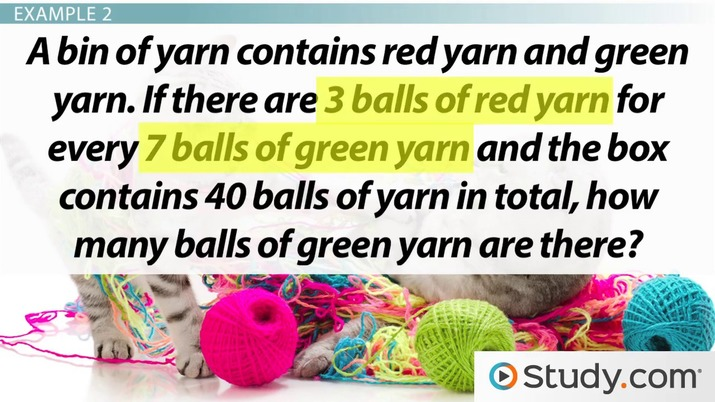# Practice Problems for Calculating Ratios and Proportions

Lesson Transcript
Instructor: Elizabeth Foster

Elizabeth has been involved with tutoring since high school and has a B.A. in Classics.

In this lesson, you'll get a quick review of a type of math problem that shows up on the SAT: ratios and proportions. We'll walk through some problems in the video, and you can test your skills in the quiz questions.

## Proportions & Ratios

This lesson is mostly dedicated to practice, but let's start with a quick review of ratios and proportions. A ratio is a comparison between two different quantities. For example, if you have 4 boys and 3 girls in a room, the ratio of boys to girls is 4 to 3.

Ratios can be expressed either with fractions or with a colon.

You can reduce ratios just like fractions. So, for example, the ratio of 4:3 is the same thing as the ratio of 16:12 or the ratio of 40:30.

Ratios come in two flavors: part-to-part or part-to-whole. An example of a part-to-part ratio is the ratio of boys to girls; an example of a part-to-whole ratio is the ratio of boys to all the students in the class.

A proportion is a statement that two ratios are equal. If you know three terms in a proportion, you can always solve for the fourth. So for example, if you know that 4/3 equals 16/x, you can solve to find that x equals 12.

When you set up proportions in SAT math problems, always be careful that the ratios you're comparing are actually the same. For example, you can't set up a proportion between a part-to-part and a part-to-whole ratio.An error occurred trying to load this video.

Try refreshing the page, or contact customer support.

Coming up next: Solving Problems Using Rates

### You're on a roll. Keep up the good work!

Replay
Your next lesson will play in 10 seconds
• 1:30 Example 1
• 2:35 Example 2
• 4:07 Example 3
• 5:05 Lesson Summary
Save Save

Want to watch this again later?

Timeline
Autoplay
Autoplay
Speed Speed

## Example 1

Now with that out of the way, let's look at a few examples. We'll start with one that's pretty simple.

In a certain kingdom, the ratio of dragons to princesses is 5:2. If there are 12 princesses in the kingdom, how many dragons are there?

First, we'll take the information in the problem to set up a proportion. 5/2 equals d/12.

Notice how we set up the ratios of dragons over princesses on both sides - remember that you have to keep the ratios the same on both sides of the proportion.

Now we'll cross-multiply to solve for d, or the number of dragons.

We learn that the number of dragons is 30. We can check this by plugging it back in to the original proportion to see if the ratio of dragons to princesses reduces to 5/2.

That checks out: 30/12 reduces to 5/2 if you divide both top and bottom by 6. So we know we've found the correct answer.

## Example 2

Ready for one that's a little tougher?

A bin of yarn contains red yarn and green yarn. If there are 3 balls of red yarn for every 7 balls of green yarn and the box contains 40 balls of yarn in total, how many balls of green yarn are there?

First, we'll take the information from the problem to set up our ratio. We know the ratio of red to green is 3:7. We also know that the total number of balls of yarn of both colors is 40.

Here we need to be very careful to avoid mixing up part-to-part and part-to-whole. The 3:7 ratio is a part-to-part comparison, but the number 40 is describing the whole. We cannot set up a proportion comparing unlike quantities!

In order to get something that we can compare to the 40, we need another ratio of part-to-whole.

This isn't hard to get, though.

To unlock this lesson you must be a Study.com Member.

### Register to view this lesson

Are you a student or a teacher?